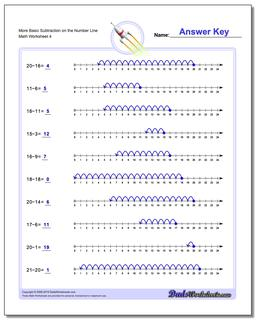# Math Worksheets: Subtraction: Subtraction: More Basic Subtraction on the Number Line (Fourth Worksheet)## More Basic Subtraction on the Number Line (Fourth Worksheet)

PropertyValue
DescriptionMore Basic Subtraction on the Number Line: These simple subtraction worksheets introduce subtraction concepts using the number Line. (Fourth Worksheet)
Resource TypeWorksheet
US Grade Level1st Grade, 2nd Grade, 3rd Grade, 4th Grade, 5th Grade, 6th Grade
Age Range6-12